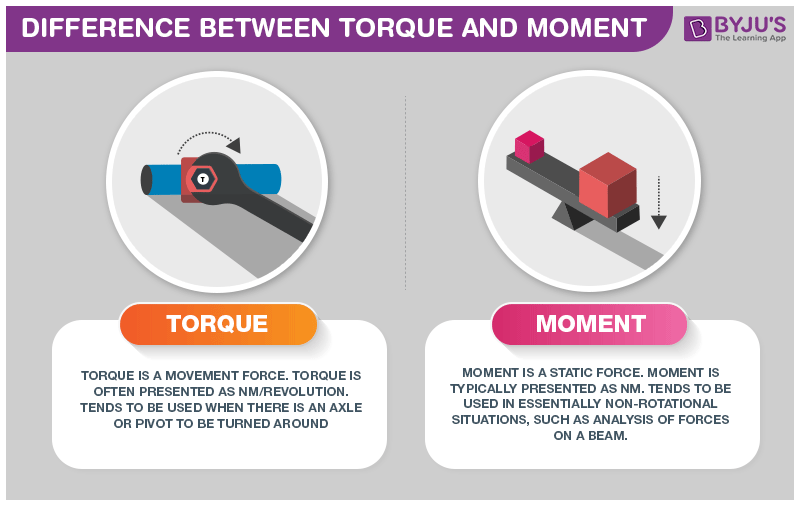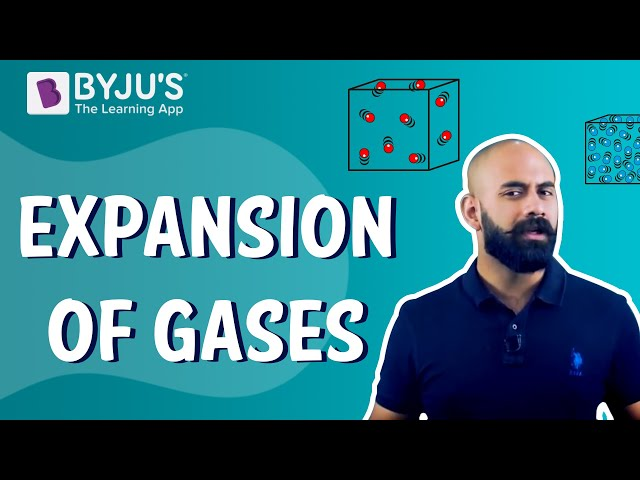Checkout JEE MAINS 2022 Question Paper Analysis : Checkout JEE MAINS 2022 Question Paper Analysis :

# Difference Between Torque and Moment## What is Torque?

Torque is the measure of force that causes an object to rotate about an axes. Just like how force causes an object to accelerate i linear kinematics, torque is what causes an object to acquire angular acceleration.

## What is Moment?

In physics, moment of force is a measure of its tendency to cause a body to rotate about a specific point or axis.

## Difference Between Torque and Moment

 Torque vs Moment Torque Moment It is the measurement of the turning force of an object It is the measurement of the perpendicular distance from the point of rotation to the force’s line of action The symbol of torque is $$\begin{array}{l}\tau\end{array}$$ and SI unit is Nm/revolution The symbol of the moment is M and SI unit if NM It is a movement-related force It is a static force Torque is used to measure the coupling The moment is not used for this purpose It is used where there is an availability of a pivot and rotation It is produced by any lateral force and used in non-rotational events It is referred to the nature of the force to rotate the object in a pivot. It is referred to the nature of the force to move the object.

These were some difference between torque and moment. If you wish to find out more, download BYJU’S The Learning App.

RELATED ARTICLES:## Frequently Asked Questions – FAQs

### What is meant by torque?

Torque is the rotational analogue of linear force. Depending on the topic, it is also termed the moment of force, the turning effect, or the rotational force.

### What is the symbol used to denote torque?

The symbol of torque is
$$\begin{array}{l}\tau \end{array}$$
.

### What is meant by moment?

In physics, the moment of force is a measure of its tendency to cause a body to rotate about a specific point or axis.

### What is the symbol used to denote a moment?

The symbol of the moment is M.

### What is the main difference between torque and moment?

Torque is the measurement of the turning force of a body, while the moment is the measurement of the perpendicular distance from the point of rotation to the force’s line of action.
Test your knowledge on Torque and moment difference

#### 1 Comment

1. Aloore Jugnuu

Great performance